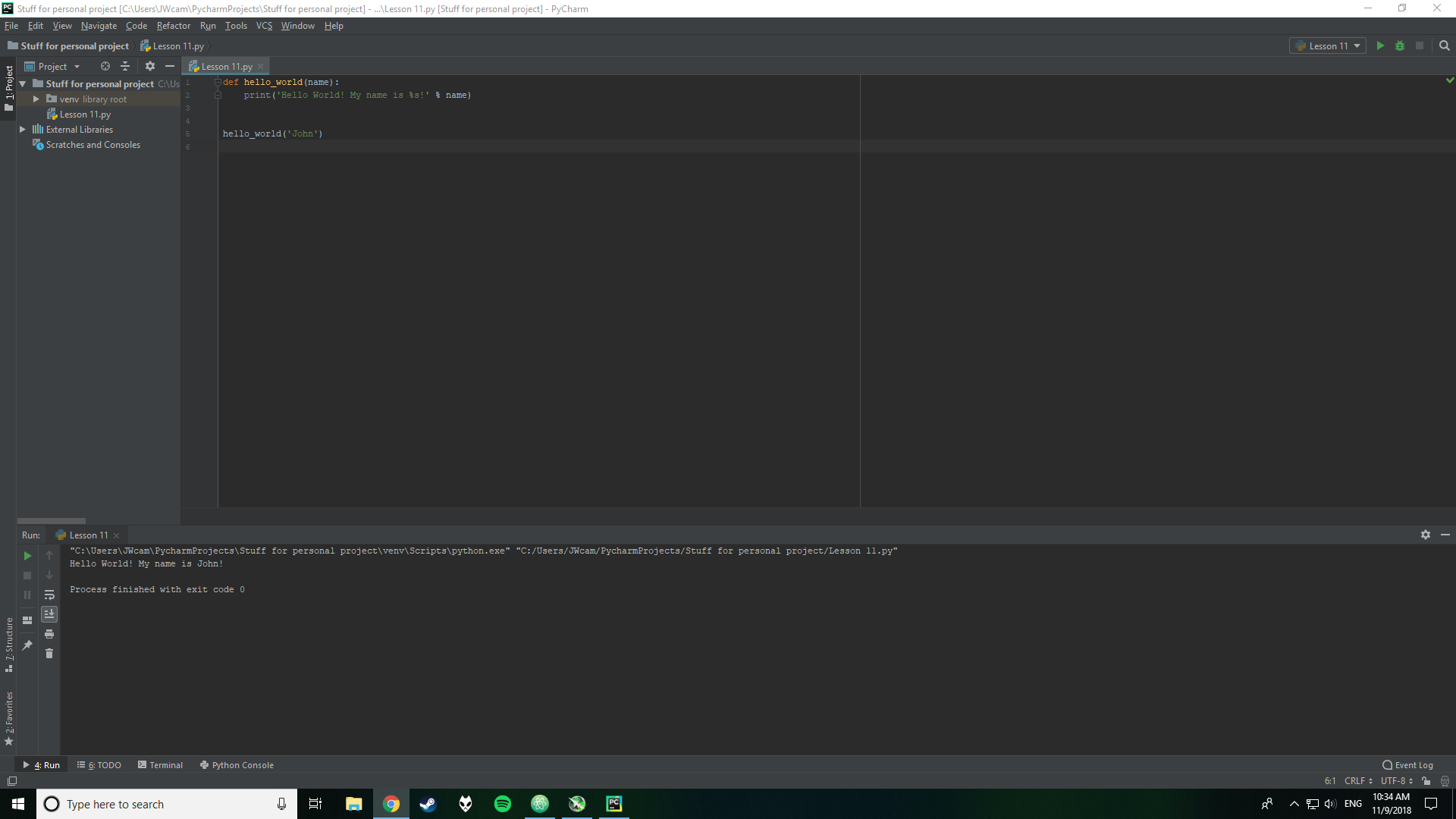# Lesson 11 - For Functions

Functions are possibly the most important concept that you will learn for not only Python, but also other programming languages that you will learn throughout your career.

Functions are used in Python to perform tasks, ranging from simple to very complex, such as checking to see if a number is even, to being used in Youtube. Each piece of code that does something in a function is called an expression.

The basic syntax for a function is the following:

def [the name of the function]([names of the arguments, don't worry I'll explain what that is in a minute]): do something with the arguments

And don't forget that you have to call the function as well, by using the following syntax: the name of the function(the values to be passed for the arguments)

An example of a function that will take the name that is passed to (given to) by the programmer, and print out Hello World! My name is [name].Sometimes you have to run the code inside of a print() to print out the results, but since our function already has a print(), it is not necessary

We used the string formatting that we learned in lesson 7, where the %s is replaced by a string, which in our case was the name of the programmer that passed it to the function

We can use for loops that we learned last lesson in conjunction with functions as well.

The use cases for for loops and functions are vast, but for now we will just use it to count.

def how_many_times(times): for x in range(1, times+1): print('I have ran this code this %d many times' % x) how_many_times(10)

Can you guess what this code would print out? Guess and check the solution to see if you were right! (side not I had to change the range to start at 1 and end 1 after since ranges usually start at 0))